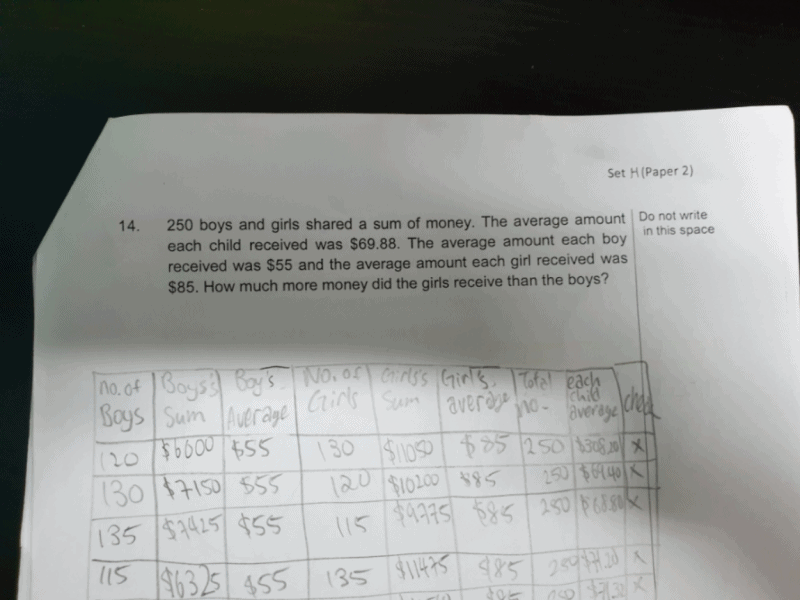# QuestionSince girls on average get more money than boys, we shall assume that all the 250 children are girls. By doing that, we get \$21 250.

However, that is more than the amount of all children which is \$17 470. So, we have to subtract \$17 470 from \$21 250, we get \$3780.

Then, we have to find the difference between the amount of all boys and all girls on average, which is \$30.

We have to divide the excess amount of \$3780 by \$30, from which we get 126, which represents the number of boys.

Now, we are able to find how much in total the boys received, which is \$6930.

With this, we subtract \$6930 from \$17470 in order to find how much in total the girls received, which is \$10 540.

We are now able to get the answer by finding the difference between girls and boys, which is \$3610, the answer.

0 Replies 0 Likes